# Reverse VLOOKUP – Right to Left – Excel & Google Sheets

This tutorial will demonstrate how to perform a “reverse VLOOKUP” in Excel and Google Sheets.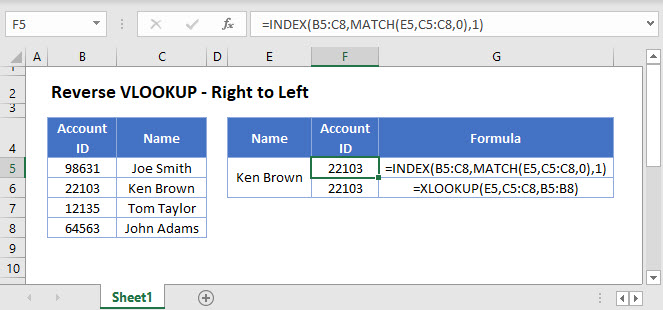## Reverse VLOOKUP – Right to Left

When you use the VLOOKUP function, the lookup column must be the left-most column in your data set. So you can only perform a lookup left to right.

However, you can work around this limitation by using the INDEX / MATCH combination (or the new XLOOKUP Function as you’ll see below).

``=INDEX(B3:D6,MATCH(F3,C3:C6,0),1)``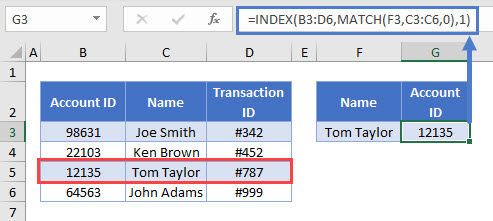The formula works as follows:

## MATCH Function

We use the MATCH function to return the relative position (i.e., the row number in the array) of the lookup item.

``=MATCH(F3,C3:C6,0)``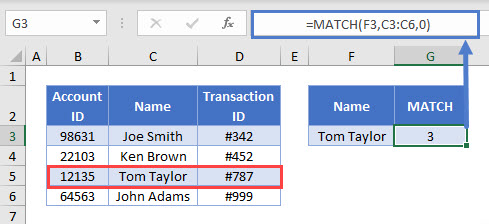Think of the MATCH Function, as similar to a VLOOKUP performed on a single column, except instead of returning the value, the row number is returned instead.

## INDEX Function

Once we have the row number, we use the INDEX Function to return the value from that row number.

``=INDEX(array, row_num,[column_num])``
``=INDEX(B3:D6,G3,1)``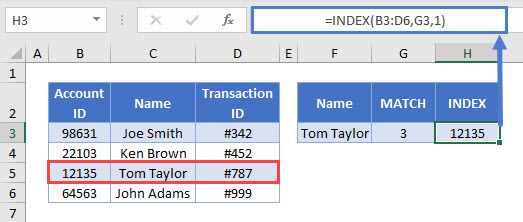The INDEX Function returns the cell value of a certain row and column in your range. The MATCH Function supplies the row number. We set the column number to be 1 because we want the first column of data.

Combining the functions, gives us our original formula:

``=INDEX(B3:D6,MATCH(F3,C3:C6,0),1)``

## XLOOKUP Function

The XLOOKUP Function was introduced after Excel 2019 to Office 365 users. The XLOOKUP Function also allows you to perform a reverse lookup:

``=XLOOKUP(F3,C3:C6,B3:B6)``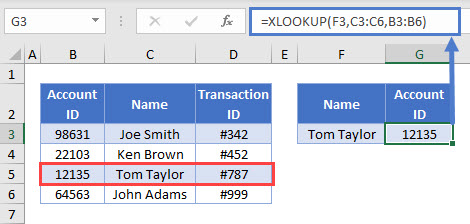## Reverse VLOOKUP Right To Left in Google Sheets

These formulas work exactly the same in Google Sheets as in Excel.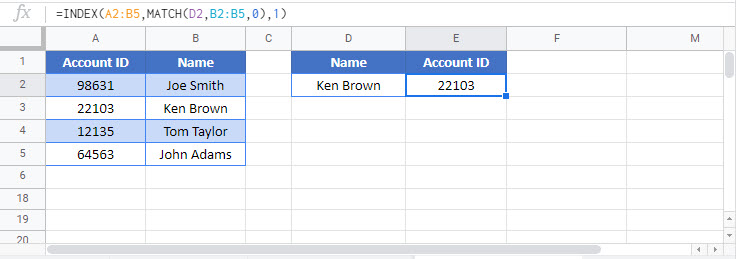### Excel Practice Worksheet

Practice Excel functions and formulas with our 100% free practice worksheets!